# Area + perimeter - math problems

#### Number of problems found: 213

• The ratio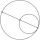The ratio of the lengths of the two circles is 5: 2. Find the ratio of a a) the radii of these circles b) the areas for these circles
• ABS trianglePoint S is the center of the square ABCD. The ABS triangle has a content of 9 cm2. What is the circumference of the square in cm?
• Wooden prism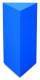Find the weight of a wooden regular triangular prism with a height equal to the perimeter of the base and a figure inscribed in a circle with a radius of 6, M cm, where M is the month of your birth. The density of oak is 680 kg/m3.
• Trapezoid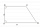The rectangular trapezoid ABCD with right angle at the vertex A has sides a, b, c, d. Calculate the circumference and the area of the trapezoid if given: a = 25cm, c = 10cm, d = 8cm
• What is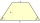What is the circumference of an isosceles trapezoid with a content of 106.75 cm 2 , the lengths of the sides are in the ratio 1: 3: 2: 1 and the bases are 6.1 cm apart?
• 144 stepsTo get around the garden we have to take 144 steps. 8 steps less in width than in length. One step is 5 decimetres long. What are the dimensions of the garden? What is its perimeter and its area?
• The isoscelesThe isosceles trapezoid ABCD has bases of 18 cm and 12 cm. The angle at apex A is 60°. What is the circumference and content of the trapezoid?
• Matilda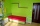Matilda measures the perimeter of her bedroom to determine how many feet of border she needs to go around the tops of the walls. Two walls are each 96 inches long, and two walls are each 3 yards long. She pays \$30 for border that costs \$1 per foot. What a
• A rectangular 4A rectangular garden has a length of 57 m and a width of 42 m. Calculate of how many m2 will decrease the area of a garden, if the ornamental fence with a width of 60 cm will be planted inside its perimeter.
• Perimeter to area 2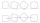Find the area of a square whose perimeter is 100 cm with solve.
• Polygon - area coordinatesFind the perimeter and the area of the polygon with the given vertices. T (2,7), U (2,9), V (5,9), W (5,7)
• Area and perimeter 2Find the area and the perimeter of a rectangle of length 45 1/2 cm and breadth 16 2/3 cm.
• Calculate triangleIn the triangle ABC, calculate the sizes of all heights, angles, perimeters and its area, if given a-40cm, b-57cm, c-59cm
• Perimeter of rhombus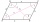The area of the rhombus is 32 cm2. One side of it measures 10 cm. The height on the other side measures 5 cm. What is the circumference of this rhombus?
• Dodecagon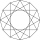Find the area of a regular dodecagon (n=12) if the radius of the circumscribed circle is 5 cm.
• Angle of diagonalsCalculate the perimeter and the area of a rectangle if its diagonal is 14 cm and the diagonals form an angle of 130°.
• A isoscelesA isosceles triangle has an area of 168 cm2 and it's added height and base is 370 cm. What are the measurements of it's height and base?
• Side and diagonalFind the circumference and the area of the rectangle if given: side a = 8 cm diagonal u = 10 cm.
• A rectangle 4A rectangle has area 300 and perimeter 80. what is the ratio of the length and width?
• Harry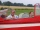Harry Thomson bought a large land in the shape of a rectangle with a circumference of 90 meters. He divided it into three rectangular plots. The shorter side has all three plots of equal length, their longer sides are three consecutive natural numbers. Fi

Do you have an exciting math question or word problem that you can't solve? Ask a question or post a math problem, and we can try to solve it.

We will send a solution to your e-mail address. Solved examples are also published here. Please enter the e-mail correctly and check whether you don't have a full mailbox.

Area - math problems. Perimeter - math problems.